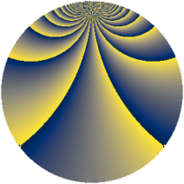# Properties

 Label 304.2.vLevel $304$ Weight $2$ Character orbit 304.v Rep. character $\chi_{304}(45,\cdot)$ Character field $\Q(\zeta_{12})$ Dimension $152$ Newform subspaces $1$ Sturm bound $80$ Trace bound $0$

# Related objects

## Defining parameters

 Level: $$N$$ $$=$$ $$304 = 2^{4} \cdot 19$$ Weight: $$k$$ $$=$$ $$2$$ Character orbit: $$[\chi]$$ $$=$$ 304.v (of order $$12$$ and degree $$4$$) Character conductor: $$\operatorname{cond}(\chi)$$ $$=$$ $$304$$ Character field: $$\Q(\zeta_{12})$$ Newform subspaces: $$1$$ Sturm bound: $$80$$ Trace bound: $$0$$

## Dimensions

The following table gives the dimensions of various subspaces of $$M_{2}(304, [\chi])$$.

Total New Old
Modular forms 168 168 0
Cusp forms 152 152 0
Eisenstein series 16 16 0

## Trace form

 $$152q - 2q^{2} - 2q^{3} - 2q^{4} - 2q^{5} - 2q^{6} - 20q^{8} + O(q^{10})$$ $$152q - 2q^{2} - 2q^{3} - 2q^{4} - 2q^{5} - 2q^{6} - 20q^{8} - 8q^{11} - 8q^{12} - 2q^{13} + 2q^{14} - 20q^{15} + 2q^{16} - 4q^{17} - 16q^{18} - 12q^{19} - 36q^{20} + 4q^{21} - 2q^{22} - 10q^{24} + 44q^{26} - 20q^{27} + 26q^{28} - 2q^{29} + 56q^{30} - 64q^{31} + 28q^{32} - 4q^{33} - 12q^{34} + 32q^{35} - 2q^{36} - 8q^{37} + 18q^{38} - 12q^{40} - 10q^{42} - 2q^{43} - 52q^{44} - 16q^{45} + 24q^{46} - 4q^{47} - 42q^{48} - 120q^{49} - 60q^{50} + 2q^{51} - 18q^{52} - 2q^{53} - 64q^{54} + 60q^{56} - 36q^{58} - 2q^{59} + 48q^{60} + 30q^{61} - 38q^{62} + 24q^{63} + 52q^{64} - 16q^{65} + 90q^{66} - 26q^{67} - 8q^{68} - 84q^{69} - 72q^{70} + 42q^{72} + 6q^{74} + 112q^{75} + 30q^{76} + 48q^{77} + 26q^{78} - 12q^{79} + 6q^{80} + 40q^{81} - 70q^{82} - 48q^{83} + 4q^{84} + 34q^{85} + 30q^{86} + 12q^{88} - 50q^{90} + 12q^{91} - 30q^{92} - 8q^{93} - 40q^{94} - 32q^{95} - 288q^{96} - 4q^{97} + 74q^{98} + 60q^{99} + O(q^{100})$$

## Decomposition of $$S_{2}^{\mathrm{new}}(304, [\chi])$$ into newform subspaces

Label Dim. $$A$$ Field CM Traces $q$-expansion
$$a_2$$ $$a_3$$ $$a_5$$ $$a_7$$
304.2.v.a $$152$$ $$2.427$$ None $$-2$$ $$-2$$ $$-2$$ $$0$$